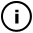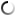# Monday, November 23, 2009

## sliding colors

int t=0;
float r1=random(-0.2,0.2);
float r2=random(-0.2,0.2);
float r3=random(-0.2,0.2);
float g1=random(-0.2,0.2);
float g2=random(-0.2,0.2);
float g3=random(-0.2,0.2);
float b1=random(-0.2,0.2);
float b2=random(-0.2,0.2);
float b3=random(-0.2,0.2);

void setup(){
size(400,200);
background(0);
}

void draw(){
++t;
for (int i=0; i<=width; ++i) {
int red=255*0.25*(2+sin(i*r1)*0.5+sin((i-t)*r2)*0.5+cos(t*r3-1.4));
int green=255*0.25*(2+sin(i*g1)*0.5+sin((i-t)*g2)*0.5+cos(t*g3-1.1));
int blue=255*0.25*(2+sin(i*b1)*0.5+sin((i-t)*b2)*0.5+cos(t*b3-0.5));
stroke(red,green,blue);
line(i,0,i,height);
}
}

## infosubmitted by: conroy
views: 2008
Sliding color bars appear to move past each other. Parameters are set randomly, so reload for a different experience.

Tags: color, trigonometry

## commentsloading...